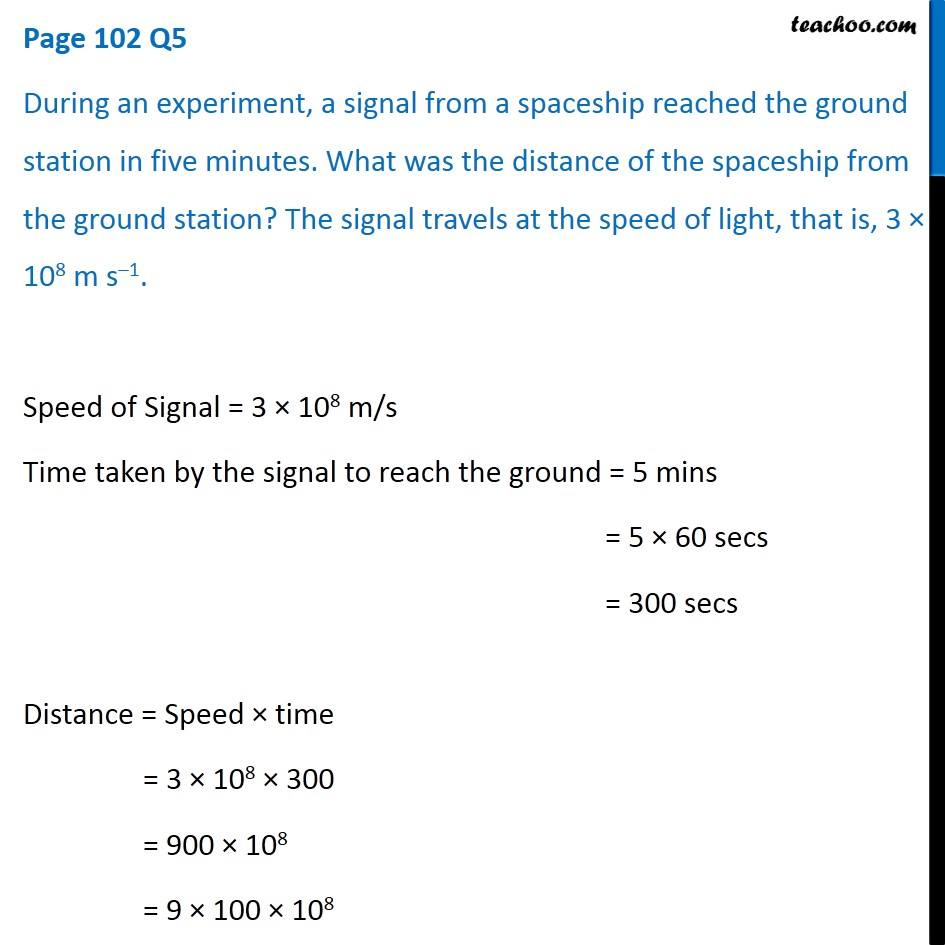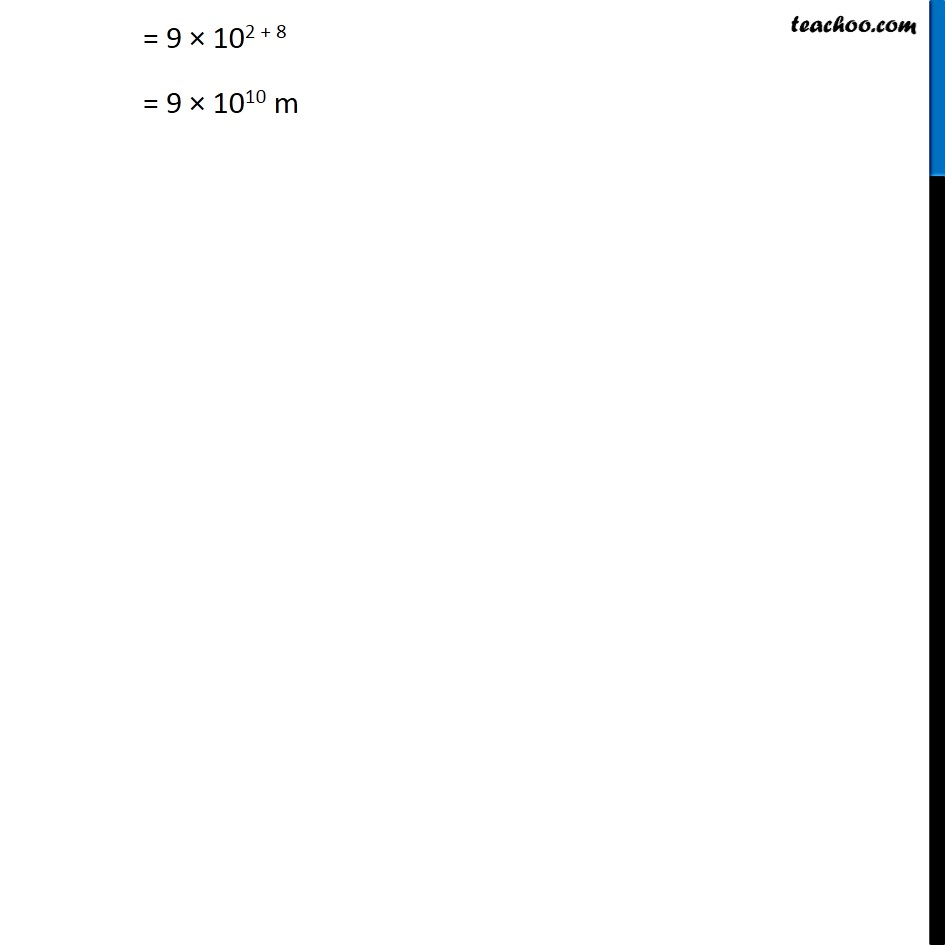Questions from Inside the chapter

Class 9
Chapter 8 Class 9 - MotionLearn in your speed, with individual attention - Teachoo Maths 1-on-1 Class

### Transcript

Page 102 Q5 During an experiment, a signal from a spaceship reached the ground station in five minutes. What was the distance of the spaceship from the ground station? The signal travels at the speed of light, that is, 3 × 108 m s–1. Speed of Signal = 3 × 108 m/s Time taken by the signal to reach the ground = 5 mins = 5 × 60 secs = 300 secs Distance = Speed × time = 3 × 108 × 300 = 900 × 108 = 9 × 100 × 108 = 9 × 102 + 8 = 9 × 1010 m